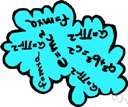abelian group

(redirected from Finite abelian group)
Also found in: Thesaurus, Encyclopedia.
Related to Finite abelian group: Fundamental theorem of finite abelian groups

a·be·li·an group

(ə-bē′lē-ən)
n.

[After Niels Henrik Abel (1802-1829), Norwegian mathematician.]

Abelian group

(əˈbiːlɪən)
n
(Mathematics) a group the defined binary operation of which is commutative: if a and b are members of an Abelian group then ab = ba
[C19: named after Niels Henrik Abel (1802–29), Norwegian mathematician]
Collins English Dictionary – Complete and Unabridged, 12th Edition 2014 © HarperCollins Publishers 1991, 1994, 1998, 2000, 2003, 2006, 2007, 2009, 2011, 2014
ThesaurusAntonymsRelated WordsSynonymsLegend:
 Noun 1Abelian group - a group that satisfies the commutative lawcommutative groupmathematical group, group - a set that is closed, associative, has an identity element and every element has an inverse
Based on WordNet 3.0, Farlex clipart collection. © 2003-2012 Princeton University, Farlex Inc.
Translations
Mentioned in ?
References in periodicals archive ?
In the case that X is a commutative ring or a finite abelian group (written multiplicative) and f maps x into its k-th power in X, we denote G(X, f) by G(X, k).
Every finite Abelian group is determined by its endomorphism monoid in the class of all groups.
Let H be a finite abelian group written additively and End(H) be the endomorphism ring of H.
For a commutative ring R with identity 1 and a finite abelian group G, written additively, let R[G] denote the group ring of G over R.
The following, due to Tutte , relates the Tutte polynomial of with the number of nowhere zero flows of over a finite abelian group (which, in our case, is eq.).
Let k be a field and G be a finite abelian group. Let kcyc be the maximal cyclotomic extension of k in an algebraic closure.
Theorem 1.1 (Fundamental theorem of finite abelian groups) Any finite abelian group G can be written as a direct sum of cyclic groups in the following canonical way: G = [MATHEMATICAL EXPRESSION NOT REPRODUCIBLE IN ASCII], where every [k.sub.i] (1 [less than or equal to] i [less than or equal to] l) is a prime power.
Tarnauceanu, An arithmetic method of counting the subgroups of a finite abelian group, Bull.
Every finite Abelian group is determined by its endomorphism semigroup in the class of all groups.
The critical group of G is a finite abelian group K(G) whose cardinality is the number of spanning trees of G.

Site: Follow: Share:
Open / Close